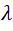# QUANTUM MECHANICS : ORIGIN, PHOTO-ELECTRIC AND COMPTON EFFECT, PRACTICE PROBLEMS AND MOCK TEST

Quantum Mechanics
is a very important part of modern physics. It give us information about the nature and behaviour of particle and system of particles at microscopic level. In this post, we will discuss about the introduction of Quantum Mechanics, Origin of Quantum Mechanics, Photo-electric effect, Compton effect, Solved numerical Problems and Practice Problems on these topics.

Introduction of Quantum Mechanics

Quantum Mechanics made up of two words, Quantum and Mechanics. Quantum means the smallest unit of any entity involved in an interaction such as energy or matter. Mechanics concern with the behaviour of particle or system of particles when subjected to forces. So, Quantum Mechanics describe the nature of microscopic particles.

Origin of Quantum Mechanics

Before 1900, the dynamics of objects or a system successfully understand with the help of Newtonian mechanics. Generally Classical Mechanics explains the things, their nature and motion at the macroscopic level. It deals with the physics of systems that can be seen by human eye. Quantum mechanics was developed as a response to the inability of classical mechanics. It explain the nature and motion of particles at atomic and sub-atomic level.

Many difficulties were encountered in explaining the following phenomena by classical mechanics -

• Photoelectric effect
• Compton effect
Quantum Mechanics originated in the year 1900 with Max Planck's explanation of the black body radiation curve. Planck assumed that the emission and absorption of electromagnetic radiations in discrete quanta having energy E is given by

where h is Planck's constant and v is the frequency. This idea of quantum utilised by Einstein to describe the phenomenon of Photo-electric Effect.

Photo-electric Effect

In 1916 Einstein studied the interaction of visible light with metals and suggested that light is not only absorbed or emitted in quanta but also propagates as quanta of energy hv. The single quanta of light are called photons.

Einstein's photoelectric equation is given as
The ultimate application of Photo-electric effect is Photovoltaic cell which convert optical energy into electric energy. There are many applications of photovoltaic cells in modern world like Burglar alarm, Fire Alarm, Television, counting machines, etc.

Compton Effect

In 1921, Prof. Compton discovered that when a beam of monochromatic radiation of high frequency is allowed to fall on a substance, capable of scattering the beam, then scattering beams contains two components of wavelength - the original wavelengthand another wavelengthwhich is larger than. The difference in wavelength of the scattered and incident rays is called compton shift.

Compton showed that -

Solved Problem-1

Problem - A Photo-electric surface has a work function of 4eV. what is the maximum velocity of the photo-electrons emitted by the light of frequencyincident on the surface?

Solution - We have the relation -

Problem - The maximum value of the change in the wavelength of the scattering light in the case of Compton scattering.

Thank You
Online Physics

1.2.3.